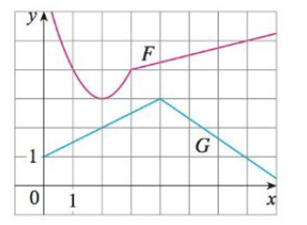Chapter 3.2, Problem 50E

Chapter
Section
Textbook Problem

# Let P(x) = F(x)G(x) and Q(x) = F(x)/G(x), where F and G are the functions whose graphs are shown.(a) Find P'(2).(b) Find Q'(7).(a)

To determine

To find: The derivative of P(x) at x=2.

Explanation

Given:

The function is P(x)=F(x)G(x).

Derivative rule: Product Rule

If f1(x) and f2(x) are both differentiable, then

ddx[f1(x)f2(x)]=f1(x)ddx[f2(x)]+f2(x)ddx[f1(x)]

Calculation:

Obtain the derivative of P(x) at x=2.

P(x)=ddx(F(x)G(x))

Apply the product rule (1) and simplify the terms,

P(x)=F(x)ddx(G(x))+G(x)ddx(F(x))=F(x)G(x)+G(x)F(x)

Substitute 2 for x in P(x),

P(x)=F(x)G(x)+G(x)F(x) (1)

From the given graph, it is observed that F(2)=3 and G(2)=2, the function F(x) is horizontal tangent at x=2 and the function G(x) is linear that passes through the points (0,1) and (4,3)

(b)

To determine

To find: The derivative of Q(x) at x=7.

### Still sussing out bartleby?

Check out a sample textbook solution.

See a sample solution

#### The Solution to Your Study Problems

Bartleby provides explanations to thousands of textbook problems written by our experts, many with advanced degrees!

Get Started

## Additional Math Solutions

#### Prove the identity. 48. tan2 sin2 = tan2 sin2

Single Variable Calculus: Early Transcendentals, Volume I

#### 30. If, find the following.

Mathematical Applications for the Management, Life, and Social Sciences

#### Evaluate 6 8 24 30

Study Guide for Stewart's Multivariable Calculus, 8th

#### In Exercises 1-22, evaluate the given expression. P(k,2)

Finite Mathematics for the Managerial, Life, and Social Sciences